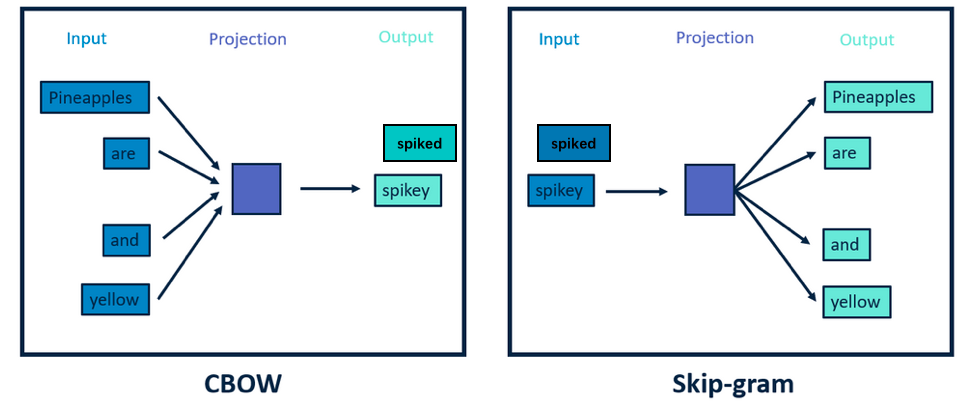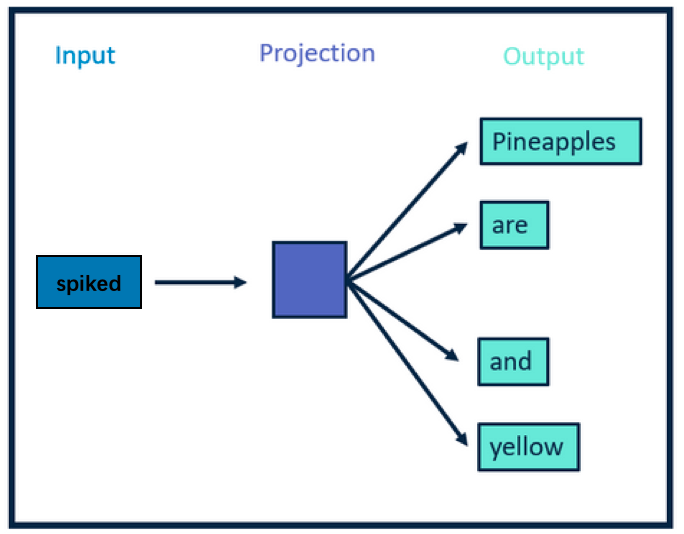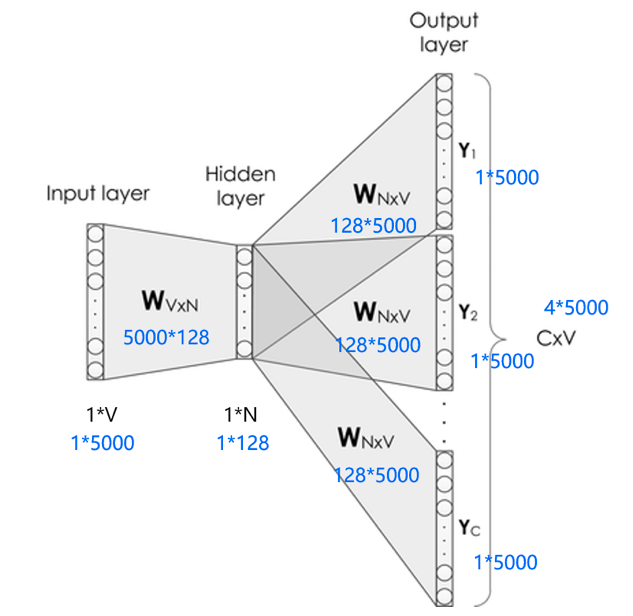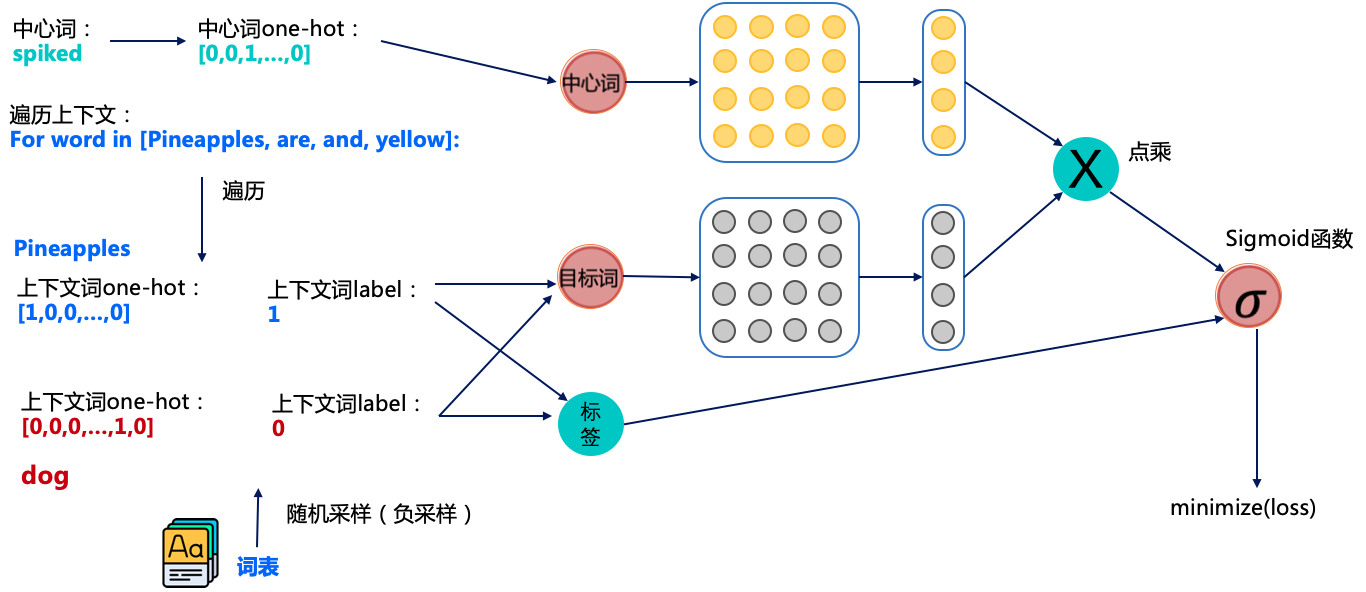# Word2Vec: 一种词向量的训练方法¶

Word2Vec本身就是在建模这个单词预测任务，当这个单词预测任务训练完成之后，那每个单词对应的词向量也就训练好了。下边我们来具体看看吧。

## 1. Word2Vec概述¶## 2. Skip-gram训练词向量原理¶## 3. Skip-gram网络结构¶Skip-gram的网络结构共包含三层：输入层，隐藏层和输出层。它的处理步骤是这样的：

1. 输入层接收shape为$$[1,V]$$的one-hot向量$$x$$，其中$$V$$代表词表中单词的数量，这个one-hot向量就是上边提到的中心词。

2. 隐藏层包含一个shape为$$[V,N]$$的参数矩阵$$W_1$$，其中这个$$N$$代表词向量的维度，$$W_1$$就是word embedding 矩阵，即我们要学习的词向量。将输入的one-hot向量$$x$$$$W_1$$相乘，便可得到一个shape为$$[1, N]$$的向量，即该输入单词对应的词向量$$e$$

3. 输出层包含一个shape为$$[N,V]$$的参数矩阵$$W_2$$，将隐藏层输出的$$e$$$$W_2$$相乘，便可以得到shape为$$[1,V]$$的向量$$r$$，内部的数值分别代表每个候选词的打分，使用softmax函数，对这些打分进行归一化，即得到中心词的预测各个单词的概率。

1. 这个输入向量是个one-hot编码的方式，只有一个元素为1，其他全是0，是个极其稀疏的向量，假设它第2个位置为1，它和word embedding相乘，便可获得word embedding矩阵的第二行的数据。那么我们知道这个规律，直接通过访存的方式直接获取就可以了，不需要进行矩阵相乘。

2. 在获取了输入单词对应的词向量$$e$$后，它是一个$$[1,N]$$向量。接下来，会使用这个向量和另外一个大的矩阵$$W_2$$进行相乘，最终会获得一个1*V的向量，然后对这个向量进行softmax，可以看到这个向量具有词表的长度，对这么长的向量进行softmax本身也是一个极其消耗资源的事情。

## 4. 负采样解决大规模分类问题¶1. 获取中心词spiked的正负样本（正负样本是目标词），这里一般会设定个固定的窗口，比如中心词前后3个词算是中心词的上下文(即正样本)；

2. 获取对应词的词向量，其中中心词从黄色的向量矩阵中获取词向量，目标词从灰色的向量矩阵中获取词向量。

3. 将中心词和目标词的词向量进行点积并经过sigmoid函数，我们知道sigmoid是可以用于2分类的函数，通过这种方式来预测中心词和目标词是否具有上下文关系。

4. 将预测的结果和标签使用交叉熵计算损失值，并计算梯度进行反向迭代，优化参数。